How Cheenta works to ensure student success?
Explore the Back-Story

# Area of a triangle | PRMO 2017 | Question 25Try this beautiful problem from the Pre-RMO, 2017, Question 25, based on Area of a triangle.

## Area of triangles - PRMO 2017, Question 25

Let ABCD be a rectangle and let E and F be points on CD and BC respectively such that area (ADE) =16, area (CEF) =9, and area (ABF) =25. What is the area of triangle AEF?

• is 107
• is 30
• is 840
• cannot be determined from the given information

### Key Concepts

Equation

Algebra

Integers

PRMO, 2017, Question 25

Higher Algebra by Hall and Knight

## Try with Hints

Let x=AD, a=DE, y-a=EC, b=FC, x-b=FB, y=AB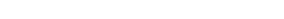or,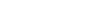or,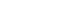or,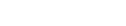or,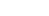or,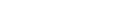or,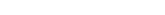or,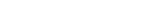or, t=80,20

or, xy=80

or, area of triangle AEF= 80-(16+9+25)=30.

## Subscribe to Cheenta at Youtube

Try this beautiful problem from the Pre-RMO, 2017, Question 25, based on Area of a triangle.

## Area of triangles - PRMO 2017, Question 25

Let ABCD be a rectangle and let E and F be points on CD and BC respectively such that area (ADE) =16, area (CEF) =9, and area (ABF) =25. What is the area of triangle AEF?

• is 107
• is 30
• is 840
• cannot be determined from the given information

### Key Concepts

Equation

Algebra

Integers

PRMO, 2017, Question 25

Higher Algebra by Hall and Knight

## Try with Hints

Let x=AD, a=DE, y-a=EC, b=FC, x-b=FB, y=ABor,or,or,or,or,or,or,or, t=80,20

or, xy=80

or, area of triangle AEF= 80-(16+9+25)=30.

## Subscribe to Cheenta at Youtube

This site uses Akismet to reduce spam. Learn how your comment data is processed.

### Knowledge Partner